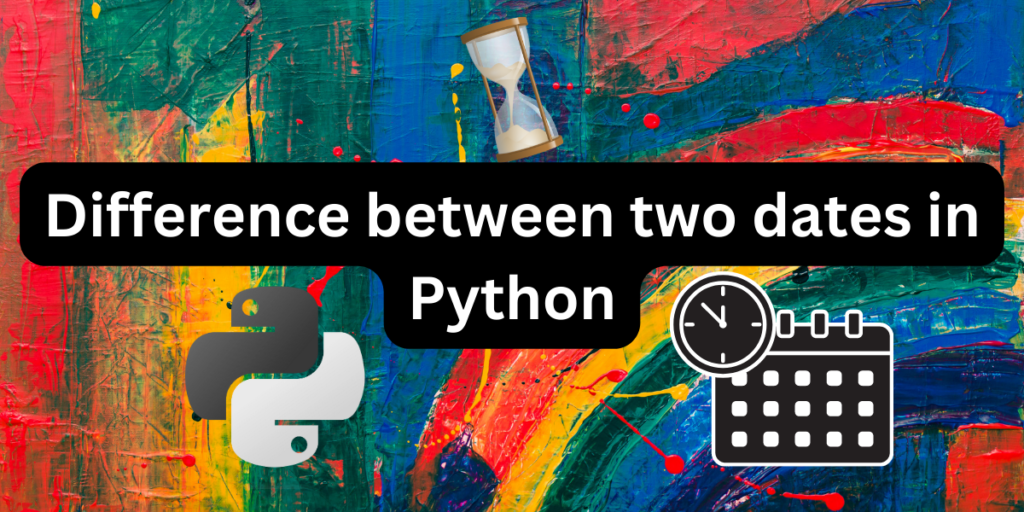# Difference between two dates in PythonThe datetime() constructor in python can be used to calculate the difference between two dates in python. We can subtract the end date from the beginning using the timedelta construct from the datetime() module in python. The timedelta construct takes into account 24 hours as one day.

When using timedelta, we need to specify the hours, minutes and seconds and set then to zero for successful computation.

Also read: Creating a range of dates in Python using datetime

Dates can be given in the form of string, date object or datetime object. In case of strings, we need to strip the string down into proper datetime format to manipulate it.

There are two main methods by which we can find the difference between two dates in python. Let’s take a look at them one by one.

To know more about datetime() visit the official documentation.

## Installing Datetime()

If you don’t already have datetime module in your system, then you need to run the following code in your command prompt in administrator mode.

```pip install datetime
```

If you’re using conda distribution instead of pip, then you’ll have to take some extra steps. You have run all the following commands in your command prompt in administrator mode.

```anaconda login
conda install -c trentonoliphant datetime
```

Apparently, anaconda sometimes have some trouble finding very common packages.

## Finding the Difference between two `date` objects

The difference between two `date`s can be directly calculated when the dates are not given as a string input. Let us look at how we can implement this:

```#importing required modules
from datetime import date
#defining the function for subtracting
def get_difference(startdate, enddate):
diff = enddate - startdate
return diff.days
#initializing dates
startdate = date(2023, 2, 20)
enddate = date(2023, 3, 15)
#storing the result and calling the function
days = get_difference(startdate, enddate)
#displaying the result
print(f'Difference is {days} days')
```

The output of the above code will be:

```Difference is 23 days
```

## Difference between two `datetime` object

In this case, there is also time involved along with a date. Here we have to strip the time from the date to calculate the days properly.

```#importing required modules
from datetime import datetime

# datetime in string format
start_date = '2023/1/20 07:15:30.365020'
end_date = '2023/2/28 05:20:45.193452'

#converting the string input to date
start_date1 = datetime.strptime(start_date, "%Y/%m/%d %H:%M:%S.%f")
end_date1 = datetime.strptime(end_date, "%Y/%m/%d %H:%M:%S.%f")

# calculating the difference in dates
diff = end_date1-start_date1
#displaying the difference
print(f'Difference is {diff.days} days')
```

The output of the above code will similar to the first one.

```Difference is 38 days
```

Also read: Convert an RGB image into grayscale using Matplotlib.

## Taking user input for date range

Now, let’s look at how we can customize the date input for each user.

```#importing required modules
import datetime

#taking user input for start date
styear = int(input('Enter start year='))
stmonth = int(input('Enter start month='))
stday = int(input('Enter start day='))
datestart = datetime.date(styear, stmonth, stday)
print("start date is=",datestart)
#taking user input for end date
endyear = int(input('Enter last year='))
endmonth = int(input('Enter last month='))
endday = int(input('Enter last day='))
dateend = datetime.date(endyear, endmonth, endday)
print("end date is=",dateend)
#defining the function for calculating difference between dates
def get_diff(startdate, enddate):
diff = enddate - startdate
return diff.days
#storing the result and calling the function
days = get_diff(datestart, dateend)
#displaying the result
print(f'Difference is {days} days')
```

The output of the above code will be:

```Enter start year=2023
Enter start month=2
Enter start day=1
start date is= 2023-02-01
Enter last year=2023
Enter last month=3
Enter last day=5
end date is= 2023-03-05
Difference is 32 days
```